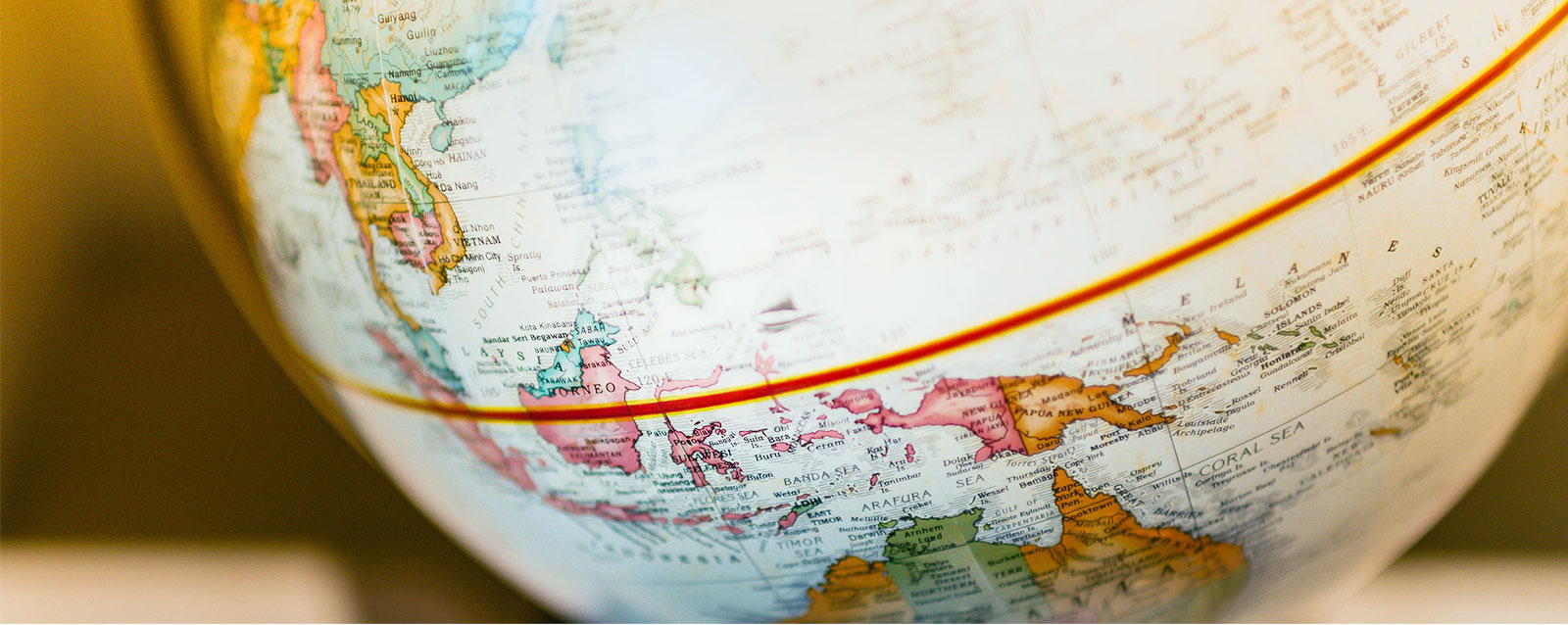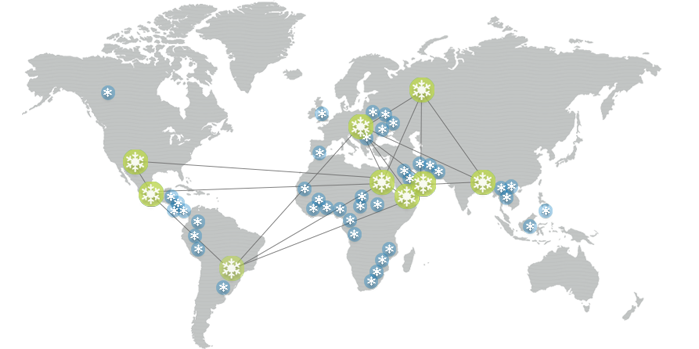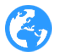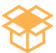### 全球足迹60+ 国家30,000+ 零售商1000+ 经销商75+ 多年的降温历史

•••••• 非洲
• 安哥拉
• 贝宁
• 布基纳法索
• 布隆迪
• 喀麦隆
• 埃及
• 埃塞俄比亚
• 加纳
• 肯尼亚
• 马里
• 莫桑比克
• 尼日利亚
• 卢旺达
• 南非
• 乌干达
• 亚洲
• 巴林
• 孟加拉国
• 塞浦路斯
• 印度
• 印度尼西亚
• 伊拉克
• 以色列
• 约旦
• 科威特
• 马来西亚
• 尼泊尔
• 阿曼
• 菲律宾
• 卡塔尔
• 沙特阿拉伯
• 斯里兰卡
• 泰国
• 土耳其
• 阿联酋
• 乌兹别克斯坦
• 越南
• 澳大利亚/大洋洲
• 澳大利亚
• 欧洲
• 阿尔巴尼亚
• 法国
• 希腊
• 爱尔兰
• 意大利
• 罗马尼亚
• 西班牙
• 特立尼达
• 英国
• 北美和南美
• 阿根廷
• 巴巴多斯
• 巴西
• 多明尼加共和国
• 墨西哥
• 巴拉圭
• 美国